Home > CCA2 > Chapter 7 > Lesson 7.2.1 > Problem7-122

7-122.
1. Solve each of the following equations. Be sure to check your solutions. Homework Help ✎

1.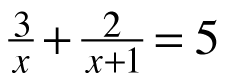2. x2 + 6x + 9 = 2x2 + 3x + 5

3.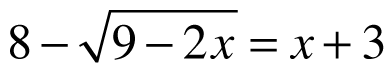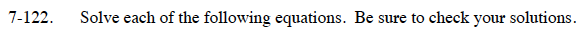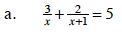Find a common denominator.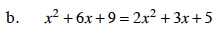Set the equation equal to zero and factor.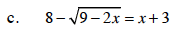Subtract 8 from both sides and then square both sides.